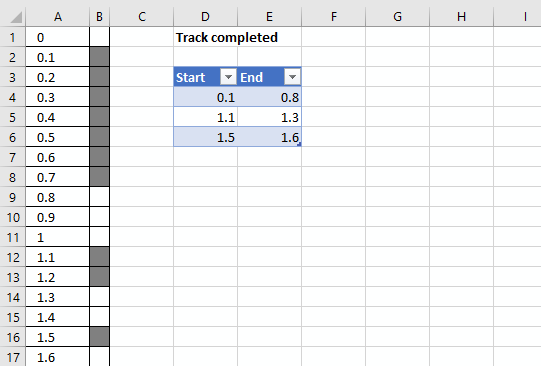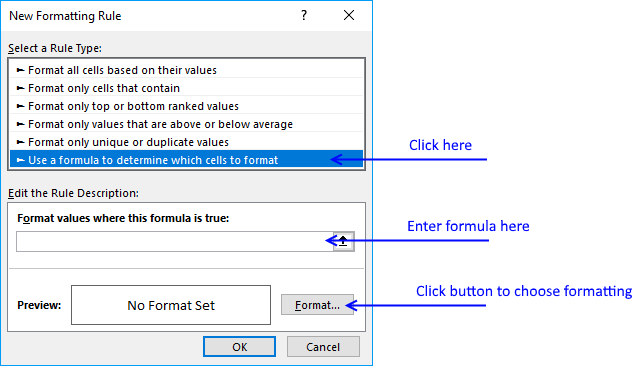Author: Oscar Cronquist Article last updated on October 02, 2018

I am working in a railway project as planner. How can i create a dynamic strip chart in excel?
Assume total length be 10 kilometers and each cell of 100 meters.
If I update the progress from 9.01 kms to 9.08 kms in the table, how the cell gets highlighted corresponding to the entered chainages.The worksheet above shows you km in column A and highlighted cells in column B, if they are complete in the excel defined table.

You can easily add or remove values to the excel defined table without the need to adjust the conditional formatting formulas.

Conditional formatting formula applied to cell range B1:B101:

=SUMPRODUCT((A1<INDIRECT("Table1[End]"))*(A1>=INDIRECT("Table1[Start]")))

#### How to build an excel table

1. Select cell range D3:E6
2. Go to tab "Insert" on the ribbon
3. Click "Table" button

Tip! You can also use shortcut key CTRL + T to create a table

Excel tables lets you easily organize, filter and format data on a worksheet.

#### How to apply a conditional formatting formula to a cell range

1. Select cell range B1:B101
2. Go to tab "Home" on the ribbon if you are not already there
3. Click on "Conditional Formatting" button to expand a menu
4. Click "New Rule.."5. Click "Use a formula to determine which cells to format"
6. Type above formula in dialog box formula bar
7. Click "Format..." button
8. Go to tab "Fill"
9. Pick a fill color
10. Click OK button
11. Click OK button

#### Explaining conditional formatting formula

You can't use structured references in a conditional formatting formula unless you use the INDIRECT function for each reference. This applies to Data Validation Lists as well.

Step 1 - Check if current cell value is less than the END range values in table

(A1<INDIRECT("Table1[End]")

becomes

0<{0.8;1.3;1.6}

and returns {TRUE;TRUE;TRUE}

Step 2 - Check if current cell value is greater than or equal to the START values in table

A1>=INDIRECT("Table1[Start]"

becomes

0>={0.1;1.1;1.5}

and returns {FALSE;FALSE;FALSE}

Step 3 - Multiply arrays

(A1<INDIRECT("Table1[End]"))*(A1>=INDIRECT("Table1[Start]"))

becomes

{TRUE;TRUE;TRUE}*{FALSE;FALSE;FALSE}

and returns {0;0;0}

Multiplying boolean values creates integers.

Step 4 - Sum array

SUMPRODUCT((A1<INDIRECT("Table1[End]"))*(A1>=INDIRECT("Table1[Start]")))

becomes

SUMPRODUCT( {0;0;0})

and returns 0.

0 means False so cell B1 is not formatted gray.